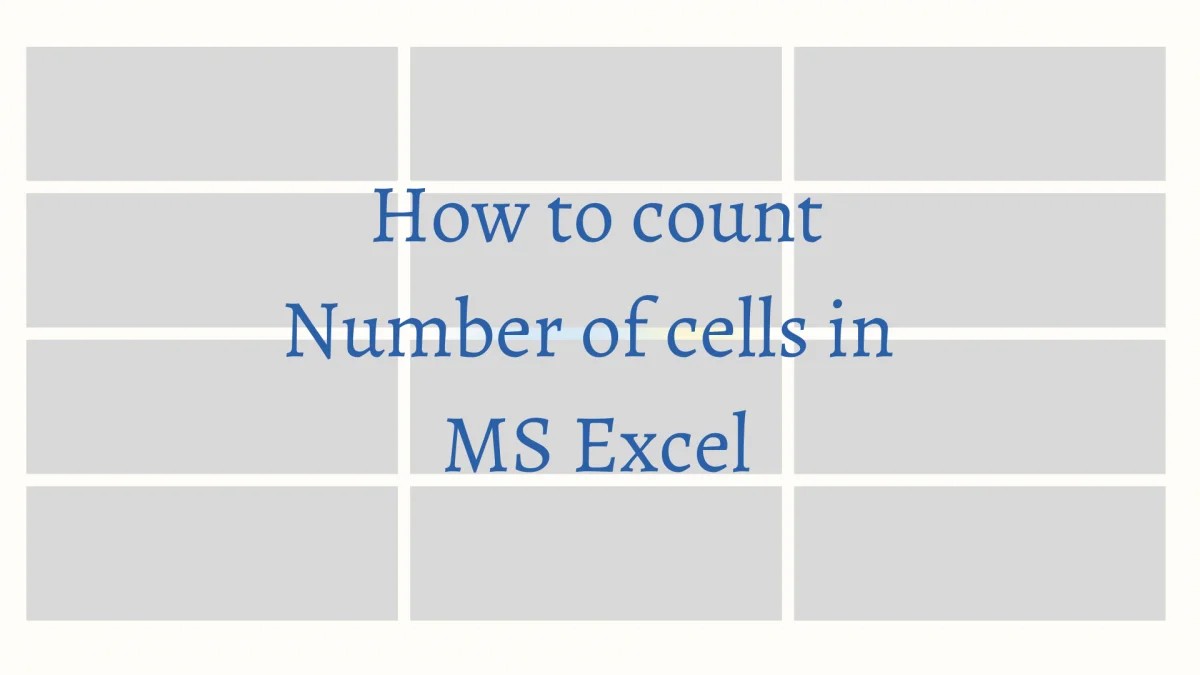# How to Count Numbers of Cells in Excel?Excel has numbers that run along from 1, all the way down to 10,48,576, but mind you, there are given for a reason – only to indicate the total numbers of rows in any sheet. Also, these numbers would also play a vital role in developing formulae to carry out any specific tasks in MS Excel.

With that being said, what if we want to count the number of cells containing data? Is there a way around doing it in MS Excel?

We are just going to look into that, using the following data in this article.

The need to count the cells may vary depending on the outcome in which one may either want to verify whether a particular count of cells is being present or to calculate the count of cells to display as a result or perhaps, to give it as a reference to the other formulae.

## Counting to Verify

In this case, we would be wanting to compare the count of the cells with the desired value to check whether we have surpassed or fallen behind.

Let’s say, we would like to know the number of columns in the above-given tabulation.

We would be needing to click on any amongst the leftmost cells, which in this case would be those belonging to column A as shown in the below image.

Now that we have clicked on cell A2 (please don’t double click, it would take you into the cell!), we would now be using the navigation keys to select the other cells.

Press the SHIFT key & without taking the finger off that key hit the right arrow key (→) until it reaches the rightmost cell of the tabulation as shown in the below image.

SHIFT + Right Arrow Key (→)

I can sense the reader asking, now that these cells have been selected, where is the count of cells. Yeah, I hear thee & here it is!

That bottom green bar would display the status of the active sheet in the workbook. So, amongst all the other default features counting the number of cells that are selected is also a part of the status bar.

So, now we have the total count of cells to verify the number of columns that are to be present in this tabulation. Now, let’s see how to display the count of cells within the worksheet.

## Counting to Calculate

In this case, we would not only be trying to count the number of cells but also make them display within a cell of the same sheet. So, let’s get on with it!

We are going to count the total number of cells filled with data in the tabulation & we have a formula that could just help us with that.

=COUNT(value1, value2…..)

The self-explanatory formula above has a syntax that we ought to understand, before diving into making use of it.

As always this formula too needs to start with an equal to sign (=) and after we type the term COUNT and open a bracket, it calls for the built-in formula in MS Excel’s repository that would count the entities.

Value 1, value2,……. given within the brackets are the cells which are to be selected. With all those clarified, we type =COUNT & select the entire tabulation of data.